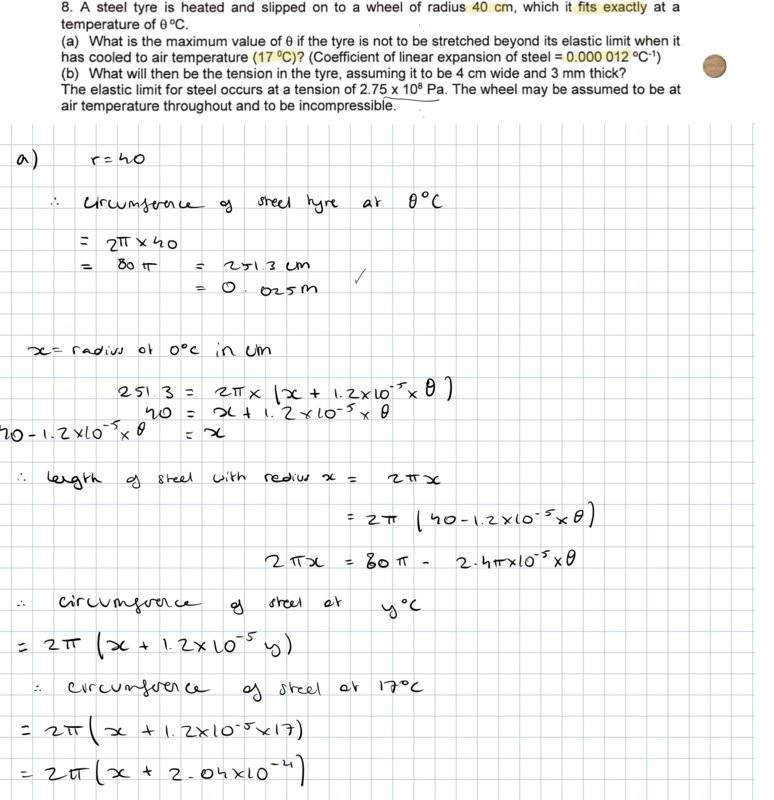# Thermal Expansion: A steel tyre is heated and slipped onto a wheel where it cools

Zararuth
Homework Statement:
8. A steel tyre is heated and slipped on to a wheel of radius 40 cm, which it fits exactly at a temperature of  oC.
(a) What is the maximum value of  if the tyre is not to be stretched beyond its elastic limit when it has cooled to air temperature (17 0C)? (Coefficient of linear expansion of steel = 0.000 012 oC-1)
(b) What will then be the tension in the tyre, assu¬ming it to be 4 cm wide and 3 mm thick?
The elastic limit for steel occurs at a tension of 2.75 x 108 Pa. The wheel may be assumed to be at air temperature throughout and to be incompressible.
Relevant Equations:
/Hi,

I have been trying to solve question A for a while now but keep getting stuck. Would anyone know what to do after this? My teacher told me that maybe I should try using the elastic limit (in part b) but normally if I had needed it, it would have been in the first part of the question.

Last edited by a moderator:

Homework Helper
Gold Member
2022 Award
if I had needed it, it would have been in the first part of the question.
From the layout I would say it is not "in" either part, but is general information to be used as necessary in either.

•DEvens and Zararuth
Homework Helper
Part b seems trivial, the tension equals the elastic limit, unless they're looking for a force rather than a stress - or unless some part of the question you may not have quoted specifies a "safety factor". I don't think you can answer part a without knowing the Young's modulus - perhaps that too is given somewhere.

•Zararuth
Mentor
Homework Statement: 8. A steel tyre is heated and slipped on to a wheel of radius 40 cm, which it fits exactly at a temperature of  oC.
(a) What is the maximum value of  if the tyre is not to be stretched beyond its elastic limit when it has cooled to air temperature (17 0C)? (Coefficient of linear expansion of steel = 0.000 012 oC-1)
(b) What will then be the tension in the tyre, assu¬ming it to be 4 cm wide and 3 mm thick?
The elastic limit for steel occurs at a tension of 2.75 x 108 Pa. The wheel may be assumed to be at air temperature throughout and to be incompressible.
Homework Equations: /

View attachment 252869
Hi,

I have been trying to solve question A for a while now but keep getting stuck. Would anyone know what to do after this? My teacher told me that maybe I should try using the elastic limit (in part b) but normally if I had needed it, it would have been in the first part of the question.
You calculated the circumference at temperature ##\theta## incorrectly. It should be $$c=2\pi (20)(1+\alpha(\theta-17))$$where ##\alpha## is the linear coefficient of thermal expansion. What is the linear strain, relative to the stain-free length at 17 C? What is the residual tensile strain after the hoop of steel cools in place back to 17 C?

•Zararuth